Journal of Vibroengineering

Published: 02 March 2021

# Effect of mistuning parameters on dynamic characteristics of mistuned bladed disk

Hongyuan Zhang1
Haocun Dong2
Tianyu Zhao3
Xin Zhang4
Huiqun Yuan5
1, 2, 4School of Automotive and Transportation, Shenyang Ligong University, Shenyang, China
3, 5School of Sciences, Northeastern University, Shenyang, China
1School of Mechanical Engineering and Automation, Northeastern University, Shenyang, China
Corresponding Author:
Haocun Dong
Views 124

#### Abstract

The aim of this paper is to study the nonlinear dynamics of the tenon and mortise of aero-engine compressor blades, based on lumped parameter model of tenon and mortise of blade disk system, considering the influence of gap and friction, The nonlinear vibration characteristics of mistuned bladed disk with different mistuning parameters are studied. The effects of tenon and mortise gap, dry friction, blade damping mistuning and tenon damping mistuning on the vibration characteristics of the mistuned bladed disk under strong coupling and weak coupling are obtained respectively. The results show that the gap and friction has little effect on the vibration response of the weakly coupled bladed disk, but the gap and friction has a great influence on the strongly coupled bladed disk, the larger the gap, the more complex the nonlinear dynamic characteristics of tenon. Under the same gap and coupling strength, the mistuning parameters have a certain influence on the vibration amplitude of the bladed disk.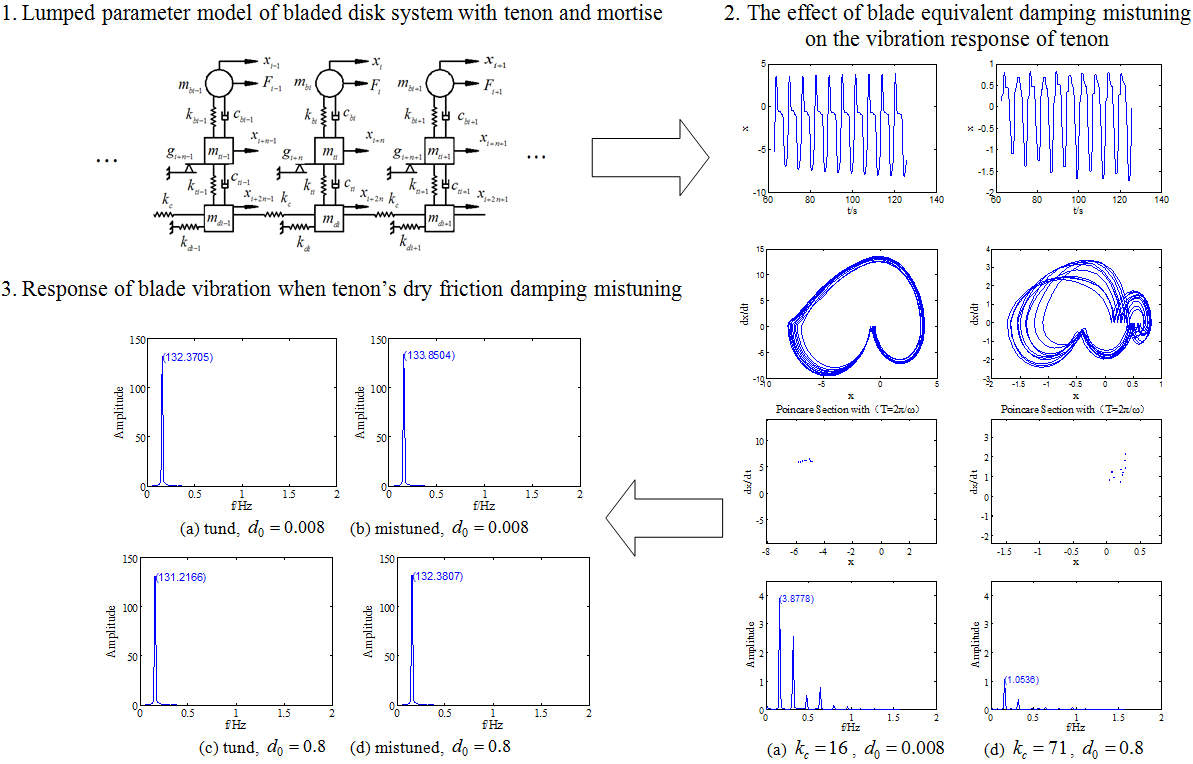#### Highlights

• A lumped parameter model of blade disk system considering the influence of gap and friction is proposed.
• The nonlinear vibration characteristics of mistuned bladed disk with different mistuning parameters are studied.
• The effects of tenon and mortise gap, dry friction, blade damping mistuning and tenon damping mistuning on the vibration characteristics of the mistuned bladed disk under strong coupling and weak coupling are obtained respectively.

## 1. Introduction

Tenon and mortise structure has the advantages of large load-bearing, small structure size and convenient maintenance and replacement. Therefore, tenon and mortise structure is widely used in aero-engine compressor and other power mechanical bladed disk system. However, due to the complexity of the working environment, the tenon and mortise structure of bladed disk system is prone to failure, The nonlinear factors such as gap and friction between tenon and mortise make the vibration characteristics of bladed disk system more complex, especially for mistuned bladed disk system.

In this paper, a lumped parameter model of the mistuned blade disk system including blade, tenon and disk is established for the compressor blade disk system of the aeroengine. The effect of the coupling stiffness, gap and friction of the tenon on the vibration characteristics of the mistuned blade disk system is considered. Blade damping mistuning and tenon damping mistuning on the vibration characteristics of the mistuned bladed disk under strong coupling and weak coupling are discussed respectively.

## 2. Dynamic model of the bladed disk system

The compressor bladed disk system of aero-engine is connected by blade and disk through tenon and mortise structure. The blade is installed on the disk through tenon. The tenon stiffness and damping are simplified as the lumped parameter dynamic model of the blade disk system with equivalent stiffness and damping, as shown in Fig. 1. Set the number of sectors to $n$, ${m}_{bi}$, ${m}_{ti}$ and ${m}_{di}$ are the equivalent mass of blade body, blade tenon and wheel disk of the $i$th sector represently, ${k}_{bi}$, ${k}_{ti}$ and ${k}_{di}$ are the equivalent stiffness of blade body, tenon and disk of the $i$th sector represents, ${c}_{bi}$ and ${c}_{ti}$ are the equivalent damping of blade body and blade tenon of the ith sector, ${F}_{i}$ is the exciting force acting on the $i$th sector, ${k}_{c}$ is the coupling stiffness between sectors. Set ${m}_{bi}$, ${m}_{ti}$ and ${m}_{di}$ displacements are ${x}_{i}$, ${x}_{i+n}$, ${x}_{i+2n}$.

When there is a small gap between tenon and mortise, the coupling effect of blade vibration in adjacent sector is variable. Set the initial gap of mortise is ${d}_{0}$, the coupling force between disks can be simplified as the elastic force of Eq. (1):

1
${f}_{g}=-{k}_{c}\left(\left|{x}_{i+n}-{x}_{i+1+n}\right|-{d}_{0}\right).$

If the tenon of the $i$th sector bears the force from the sectors on the left and right sides respectively are ${f}_{L}$ and ${f}_{R}$:

2
${f}_{L}=\left\{\begin{array}{ll}-{k}_{c}\left(\left|{x}_{i+n}-{x}_{i-1+n}\right|-{d}_{0}\right),& \left|{x}_{i+n}-{x}_{i-1+n}\right|>{d}_{0},\\ 0,& \left|{x}_{i+n}-{x}_{i-1+n}\right|\le {d}_{0},\end{array}\right\$
3
${f}_{R}=\left\{\begin{array}{ll}-{k}_{c}\left(\left|{x}_{i+n}-{x}_{i+1+n}\right|-{d}_{0}\right),& \left|{x}_{i+n}-{x}_{i+1+n}\right|>{d}_{0},\\ 0,& \left|{x}_{i+n}-{x}_{i+1+n}\right|\le {d}_{0}.\end{array}\right\$

Fig. 1Lumped parameter model of bladed disk system with tenon and mortise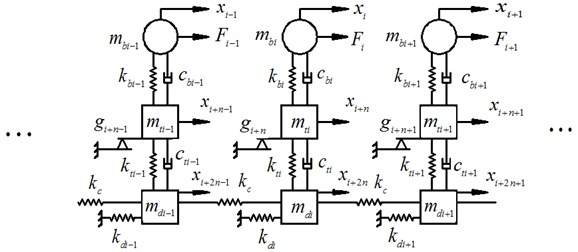The friction between the blade tenon and the disk mortise adopts the Coulomb friction model shown in Eq. (4):

4
${g}_{i+n}=-\mu {N}_{i+n}\mathrm{s}\mathrm{g}\mathrm{n}\left({\stackrel{˙}{x}}_{i+n}\right),$

where, $\mu$ is the coefficient of friction; ${N}_{i+n}$ is normal pressure; $\mathrm{s}\mathrm{g}\mathrm{n}$ is a symbolic function, when relative sliding speed ${\stackrel{˙}{x}}_{i+n}$ greater than zero, equal to zero and less than zero respectively, $\mathrm{s}\mathrm{g}\mathrm{n}$return values are 1, 0, and –1, respectively.

The exciting force of air flow acting on the $i$th blade is:

5

where is the amplitude of the exciting force on the $i$th rotor blade, $\omega$ is the excitation frequency, $n$ is the number of rotor blades, ${\varphi }_{i}$ represents the phase angle of the traveling wave excitation force on the $i$th blade, as shown in Eq. (6):

6
$\begin{array}{ll}{\varphi }_{i}=\frac{2\pi E\left(i-1\right)}{n},& i=1,2,\cdots ,n\end{array},$

where is the order of excitation.

The dynamic differential equation of the $i$th sector of the bladed disk system is obtained by analyzing the force on the $i$th sector:

7

Then:

8

The dynamic equation of the whole disk system is:

9
$\left[M\right]\left\{\stackrel{¨}{X}\right\}+\left[C\right]\left\{\stackrel{˙}{X}\right\}+\left[K\right]\left\{X\right\}=\left\{F\right\},$

where the displacement vector is $\left\{X\right\}={\left\{{x}_{1},\cdots ,{x}_{i},\cdots ,{x}_{i+n},\cdots ,{x}_{i+2n},\cdots ,{x}_{3n}\right\}}_{3n×1}^{T}$.

The force vector is $\left\{F\right\}={\left\{{F}_{1},{F}_{1},\cdots ,{F}_{n},{g}_{1+n},{g}_{2+n},\cdots ,{g}_{2n},2{k}_{c}{d}_{0},\cdots ,2{k}_{c}{d}_{0}\right\}}_{3n×1}^{T}$.

The mass matrix is:

10
$\left[M\right]={\left[\begin{array}{lll}{M}_{b}& 0& 0\\ 0& {M}_{t}& 0\\ 0& 0& {M}_{d}\end{array}\right]}_{3n×3n},$

where:

$\left[{M}_{d}\right]={\left[\begin{array}{llll}{m}_{d1}& & & \\ & {m}_{d2}& & \\ & & \ddots & \\ & & & {m}_{dn}\end{array}\right]}_{n×n}.$

The damping matrix is:

11
$\left[C\right]={\left[\begin{array}{lll}{C}_{b}& -{C}_{b}& 0\\ -{C}_{b}& {C}_{b}+{C}_{t}& -{C}_{t}\\ 0& -{C}_{t}& {C}_{t}\end{array}\right]}_{3n×3n},$

where:

The stiffness matrix is:

12
$\left[K\right]={\left[\begin{array}{ccc}{K}_{b}& -{K}_{b}& 0\\ -{K}_{b}& {K}_{b}+{K}_{t}+2{K}_{c}& -{K}_{t}\\ 0& -{K}_{t}& {K}_{t}+{K}_{d}+2{K}_{c}+{K}_{c}^{\mathrm{\text{'}}}\end{array}\right]}_{3n×3n},$

where:

$\left[{K}_{c}^{\mathrm{\text{'}}}\right]={\left[\begin{array}{ccccc}0& -{k}_{c}& 0& \cdots & 0\\ -{k}_{c}& & & & ⋮\\ 0& & \ddots & & 0\\ ⋮& & & & -{k}_{c}\\ 0& \cdots & 0& -{k}_{c}& 0\end{array}\right]}_{n×n}.$

The dimensionless parameters of disk system are defined as follows: blade body equivalent mass ${m}_{b}=\text{1}$, blade tenon equivalent mass ${m}_{t}=\text{3.75}$, equivalent mass of wheel disk ${m}_{d}=\text{3}$, blade equivalent stiffness ${k}_{b}=\text{1}$, equivalent stiffness of tenon ${k}_{t}=\text{9.1}$, equivalent stiffness of disk ${k}_{d}=\text{1.1}$, select coupling stiffness ${k}_{c}=\text{16}$ is weak coupling, coupling stiffness ${k}_{c}=\text{71}$ is strong coupling, blade equivalent damping ${c}_{b}=\text{0.05}$, equivalent damping of tenon${c}_{t}=\text{0.625}$, order of exciting force $E=\text{6}$. In order to verify the accuracy of the calculation model in this paper, Using the above parameters, through comparison with the model in reference , the comparison results are shown in Fig. 2. The horizontal and vertical coordinates are dimensionless physical quantities without units.

Fig. 2Model validation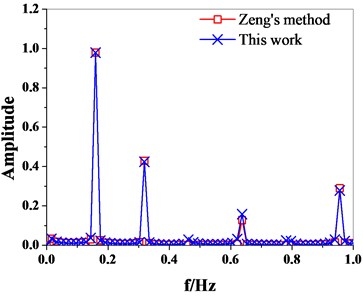It can be seen from Fig. 2 that the model in this paper is in good consistent with the model in reference , which verifies the correctness of the model in this paper.

## 3. Nonlinear dynamic analysis of the Tenon and mortise of mistuned bladed disk

### 3.1. Nonlinear vibration characteristics of tenon with dry friction

The dynamic equation of the tuned bladed disk is:

13
$\left[M\right]\left\{\stackrel{¨}{X}\right\}+\left[C\right]\left\{\stackrel{˙}{X}\right\}+\left[K\right]\left\{X\right\}=\left\{F\right\}.$

When mass, damping and stiffness are mistuning, the dynamic equation of the mistuned bladed disk is:

14
$\left[M+\mathrm{\Delta }M\right]\left\{\stackrel{¨}{X}\right\}+\left[C+\mathrm{\Delta }C\right]\left\{\stackrel{˙}{X}\right\}+\left[K+\mathrm{\Delta }K\right]\left\{X\right\}=\left\{F\right\},$

where, $M$, $C$ and $K$ are mass matrix, damping matrix and stiffness matrix respectively. $\mathrm{\Delta }M$ is the mass mistuning matrix, $\mathrm{\Delta }C$ is the damping mistuning matrix, $\mathrm{\Delta }K$ is the stiffness mistuning matrix:

15
$\left[\mathrm{\Delta }M\right]={\left[\begin{array}{llll}\mathrm{\Delta }{m}_{b1}& & & \\ & \mathrm{\Delta }{m}_{b2}& & \\ & & \ddots & \\ & & & \mathrm{\Delta }{m}_{bn}\end{array}\right]}_{n×n},$
16
$\left[\mathrm{\Delta }C\right]={\left[\begin{array}{llll}\mathrm{\Delta }{c}_{b1}& & & \\ & \mathrm{\Delta }{c}_{b2}& & \\ & & \ddots & \\ & & & \mathrm{\Delta }{c}_{bn}\end{array}\right]}_{n×n},$
17
$\left[\mathrm{\Delta }{K}_{b}\right]={\left[\begin{array}{llll}\mathrm{\Delta }{k}_{b1}& & & \\ & \mathrm{\Delta }{k}_{b2}& & \\ & & \ddots & \\ & & & \mathrm{\Delta }{k}_{bn}\end{array}\right]}_{n×n},$

where, $\mathrm{\Delta }{m}_{bi}$, $\mathrm{\Delta }{c}_{bi}$ and $\mathrm{\Delta }{k}_{bi}$ are mass mistuning, damping mistuning and stiffness mistuning of the ith blade respectively.

In this paper, the dynamic analysis of the mistuned bladed disk is carried out when only the blade stiffness mistuning is considered, and the tenon and mortise gap and friction are considered.

### 3.1.1. Vibration response of tenon without friction between Tenon and mortise

Considering that the coupling stiffness between the disks is strong coupling and weak coupling, the excitation frequency $\omega =\text{1}$ is selected, and the initial gap ${d}_{0}=\text{0.008}$ and ${d}_{0}=\text{0.8}$ are respectively. Fig. 3 shows the vibration response of tenon without friction.

When friction is not taken into account, 2-9 SHG appears in the response spectrum of the strong coupling small gap system, and the 2, 4, 7 SHG components are larger. When the coupling stiffness is weak coupling and small gap, the maximum amplitude is 4.165, and there are multiple frequency doubling phenomena in the system response spectrum, among which the second harmonic component is the largest. There are irregular points in the section of the tenon Poincare, and the waveform in time domain is random. When the coupling stiffness is strong coupling and large gap, the maximum amplitude is 1.041, and the system response spectrum appears 2-9 frequency doubling, among which 2, 4, 7 frequency components are larger. There are irregular points in the Poincare section of the tenon, and the position of the unsmooth waveform in time domain may be caused by the influence of frequency components of 2, 4 and 7. When the coupling stiffness is weak coupling and large gap, the maximum amplitude is 4.3453. There are multiple frequency doubling phenomena in the system response spectrum, among which the second frequency component is the largest. There are irregular points in the tongued Poincare section, and the time-domain waveform is random. These characteristics indicate that the system is in a chaotic state. Under the same coupling strength, when the friction is not considered, the vibration of tenon increases with the gap.

Fig. 3Response characteristics of tenon without friction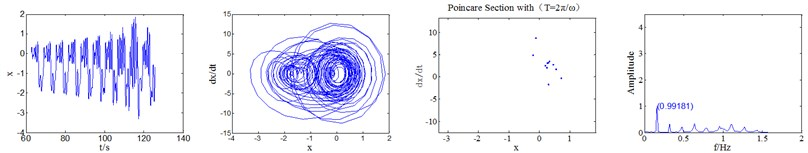a)${k}_{c}=\text{71}$, $\omega =\text{1}$, ${d}_{0}=\text{0.008}$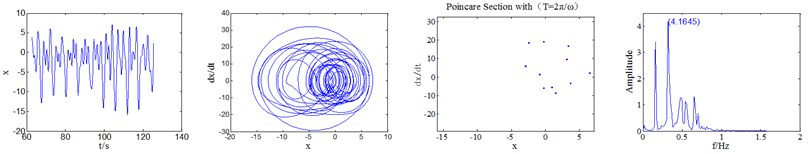b), $\omega =\text{1}$, ${d}_{0}=\text{0.008}$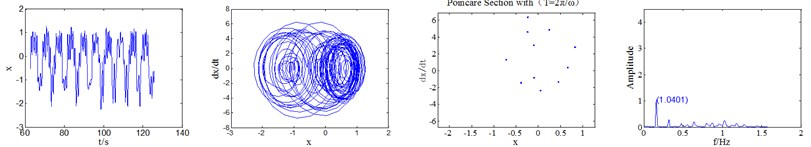c)${k}_{c}=\text{71}$, $\omega =\text{1}$, ${d}_{0}=\text{0.8}$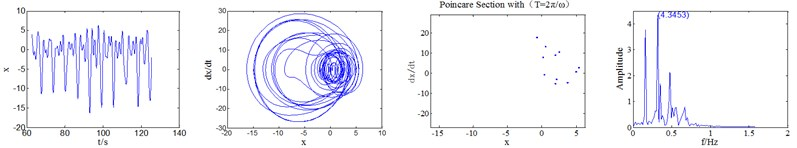d)${k}_{c}=\text{16}$, $\omega =\text{1}$, ${d}_{0}=\text{0.8}$

### 3.1.2. Vibration response of tenon with friction between Tenon and mortise

When the coupling stiffness is strong coupling, the excitation frequency $\omega =\text{1}$ and the initial gap ${d}_{0}=\text{0.008}$ are selected.

When the coupling stiffness is strong coupling and small gap, the maximum amplitude is 1.0369, and there are 2-8 frequency doubling in the system response spectrum, among which the 2, 4, 5 frequency components are larger. There are irregular points in the section of the tenon Poincare, and the position of the unsmooth time-domain waveform may be caused by the influence of the second, fourth and fifth harmonic components. When the coupling stiffness is weak coupling and small gap, the maximum amplitude is 3.7074, and there are multiple frequency doubling phenomena in the system response spectrum, among which the second, fourth and sixth harmonic components are larger. There are irregular points in the section of the tenon Poincare, and the position of the unsmooth waveform in time domain may be caused by the influence of frequency components of 2, 4 and 6. When the coupling stiffness is strong coupling and large gap, the maximum amplitude is 1.0578, there are several frequency doubling phenomena in the system response spectrum, among which the second harmonic component is the largest. There are irregular points in the section of the tenon Poincare. When the coupling stiffness is weak coupling and large gap, the maximum amplitude is 4.3453, in which 2, 3, 4 SHG components are larger. There are irregular points in the Poincare section of Tenon and mortise, and the position of unsmooth waveform in time domain may be caused by the influence of frequency components of 2, 3 and 4. These characteristics indicate that the system is in chaotic state.

From the comparison between Fig. 3 and Fig. 4, it can be seen that when the coupling stiffness is strong coupling, the vibration amplitude of tenon is smaller than that of weak coupling, and when friction is not considered, the vibration amplitude of tenon is small regardless of whether the initial gap is small or large. When the coupling stiffness is weak coupling, the vibration amplitude of tenon is larger when the friction is considered.

Fig. 4Response characteristics of tenon with friction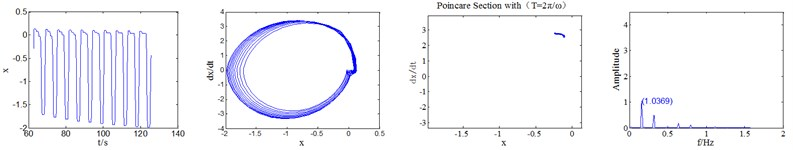a)${k}_{c}=\text{71}$, $\omega =\text{1}$, ${d}_{0}=\text{0.008}$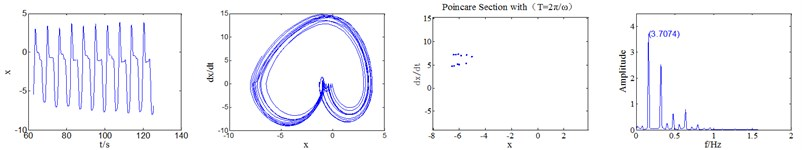b), $\omega =\text{1}$, ${d}_{0}=\text{0.008}$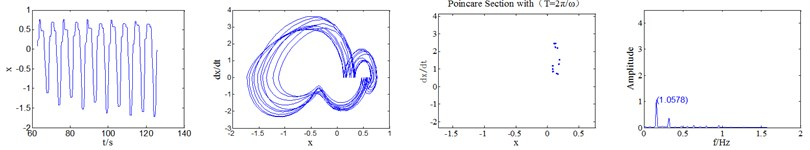c)${k}_{c}=\text{71}$, $\omega =\text{1}$, ${d}_{0}=\text{0.8}$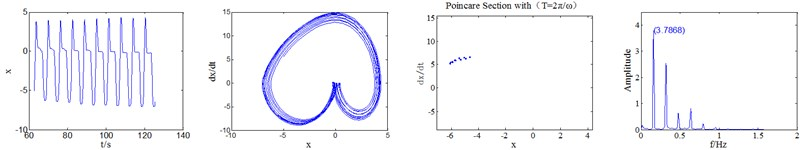d)${k}_{c}=\text{16}$, $\omega =\text{1}$, ${d}_{0}=\text{0.8}$

### 3.2. Effects of tenon gap and dry friction on blade vibration response of mistuned bladed disk

It can be seen from Fig. 5 and Fig. 6 that the maximum vibration amplitude of the mistuned blade is smaller than that without considering the friction force when the gap between tenon and groove and friction force are considered. When the coupling stiffness between disks is weak, the maximum amplitude of blades in the mistuned bladed disk system considering the friction between tenons and mortise is small. Compared with Fig. 5 and Fig. 6, it can be seen that the mistuning will change the vibration amplitude of the bladed disk system, and the existence of the mistuning factor makes the dynamic behavior of the bladed disk system more complex. The coupling strength has a great influence on the vibration amplitude of the blade, and the strong coupling makes the energy transfer between sectors strengthen, resulting in the increase of the vibration amplitude of the blade.

Fig. 5Blade vibration response amplitude of strongly coupled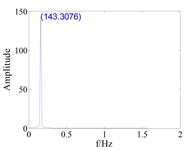a)${d}_{0}=\text{0.008}$, without friction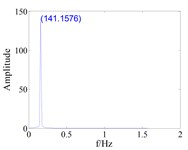b)${d}_{0}=\text{0.008}$, with friction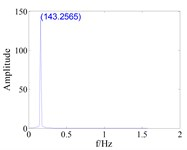c)${d}_{0}=\text{0.8}$, without friction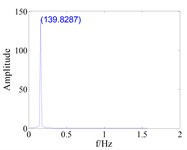d)${d}_{0}=\text{0.8}$, with friction

Fig. 6Blade vibration response amplitude of weak coupled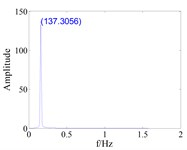a)${d}_{0}=\text{0.008}$, without friction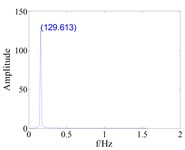b)${d}_{0}=\text{0.008}$, with friction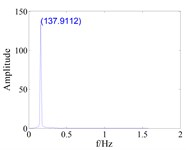c)${d}_{0}=\text{0.8}$, without friction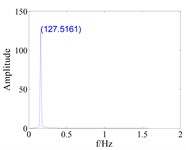d)${d}_{0}=\text{0.8}$, with friction

## 4. Effect of damping mistuning on vibration characteristics of mistuned bladed disk

### 4.1. Effect of blade equivalent damping mistuning on vibration characteristics of mistuned bladed disk

Considering the effect of blade equivalent damping mistuning on the vibration characteristics of bladed disk with different gap and coupling strength, the coupling stiffness of 16 and 71 is selected as the weak coupling and strong coupling, and the gap of 0.008 and 0.8 is selected as the small gap and large gap. Through analysis, the time domain waveform diagram, phase diagram, Poincare section and tenon frequency spectrum of tenon are obtained as shown in Fig. 7.

It can be seen from Fig. 7 that when the gap is the same, the vibration amplitude of the tenon is smaller than that of the weak coupling under strong coupling. In the case of strong coupling, when the gap is large, the vibration amplitude of the tenon is smaller than that when the gap is small. In the case of weak coupling, when the gap is large, the vibration amplitude of the tenon is larger than that of the small gap.

It can be seen from Fig. 8 and Fig. 9 that under the condition of the same gap, the vibration amplitude of the blade with strong coupling is larger; when the coupling stiffness and gap are the same, the equivalent damping mistuning of the blade body will increase the vibration amplitude of the blade, but the vibration amplitude of the blade will change slightly. The mistuning of damping leads to the enhancement of vibration energy transfer in each sector and the increase of blade vibration amplitude.

Fig. 7Effect of blade equivalent damping mistuning on vibration response of tenon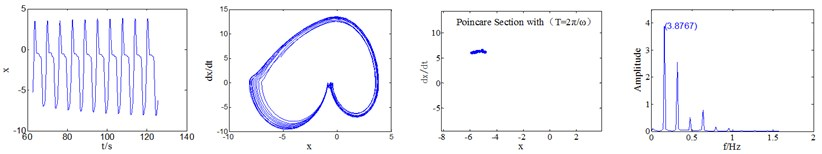a)${k}_{c}=\text{16}$, ${d}_{0}=\text{0.008}$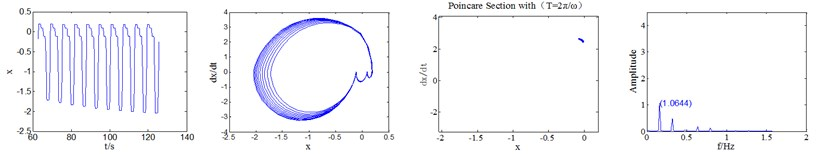b), ${d}_{0}=\text{0.008}$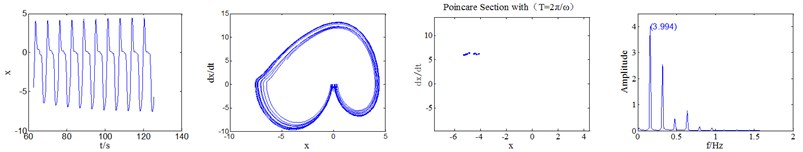c)${k}_{c}=\text{16}$, ${d}_{0}=\text{0.8}$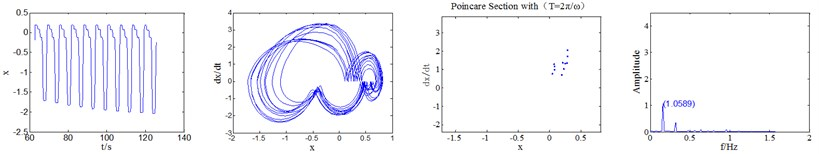d)${k}_{c}=\text{71}$, ${d}_{0}=\text{0.8}$

Fig. 8Response of blade equivalent damping mistuning to blade vibration with small gap (d0=0.008)a) Tuned, ${k}_{c}=\text{16}$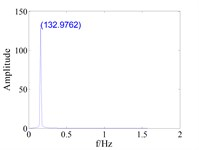b) Mistuned, ${k}_{c}=\text{16}$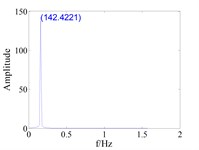c) Tuned, ${k}_{c}=\text{71}$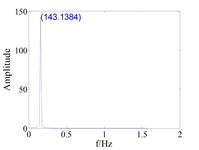d) Mistuned, ${k}_{c}=\text{71}$

Fig. 9Response of blade equivalent damping mistuning to blade vibration with large gap (d0=0.8)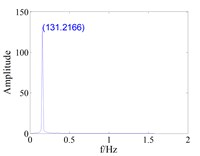a) Tuned, ${k}_{c}=\text{16}$b) Mistuned, ${k}_{c}=\text{16}$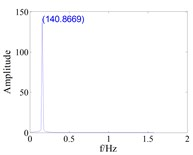c) Tuned, ${k}_{c}=\text{71}$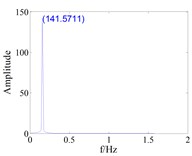d) Mistuned, ${k}_{c}=\text{71}$

### 4.2. Effect of tenon equivalent damping mistuning on vibration characteristics of mistuned bladed disk

In the case of different coupling strength and gap, the frequency spectrum of the coupling diagram with strong coupling stiffness ${k}_{c}\text{=71}$ and weak coupling stiffness ${k}_{c}=\text{16}$ are selected respectively. The gaps of 0.008 and 0.8 were selected as small and large gaps, the effect of blade equivalent damping mistuning on the vibration response of tenon is obtained by analysis.

It can be seen from Fig. 10 that when the gap is the same, the vibration amplitude of the tenon is smaller than that of the weak coupling under strong coupling; in the case of strong coupling, when the gap is large, the vibration amplitude of the tenon is smaller than that when the gap is small; in the case of weak coupling, when the gap is large, the vibration amplitude of the tenon is larger than that of the small gap. It can be seen from the comparison of Fig. 4 and Fig. 10 that under the small gap, blade equivalent damping mistuning increases the vibration amplitude of tenon.

Fig. 10The effect of blade equivalent damping mistuning on the vibration response of tenon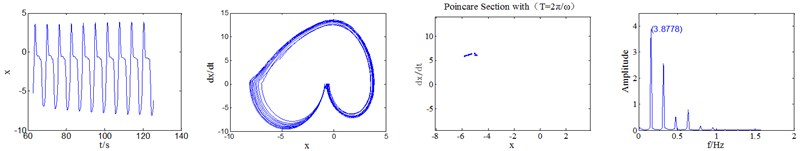a)${k}_{c}=\text{16}$, ${d}_{0}=\text{0.008}$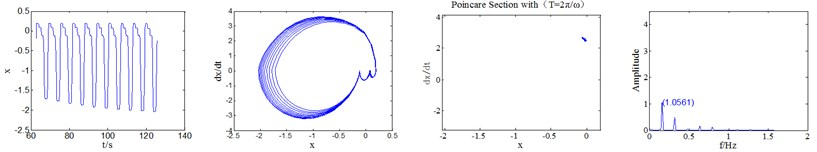b), ${d}_{0}=\text{0.008}$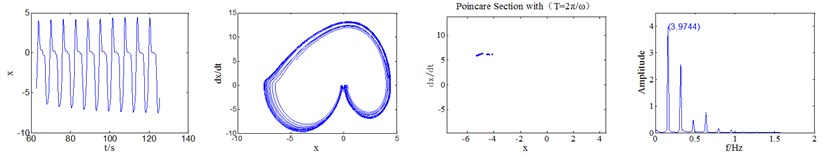c)${k}_{c}=\text{16}$, ${d}_{0}=\text{0.8}$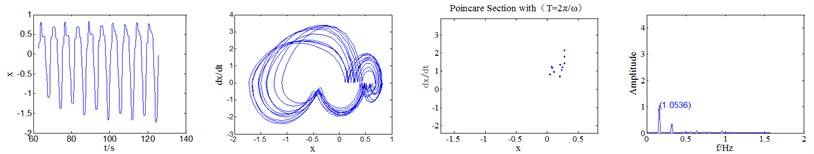d)${k}_{c}=71$, ${d}_{0}=\text{0.8}$

It can be seen from Fig. 11 and Fig. 12 that when the gap is large, the equivalent damping mistuning of the blade body will increase the vibration amplitude of the blade; when the gap is small, the equivalent damping mistuning of the blade body has little influence on the vibration amplitude of the blade.

Fig. 11The effect of tenon equivalent damping mistuning on the vibration response of blade with small gap (d0=0.008)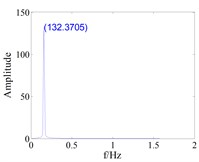a) Tuned, ${\mathrm{k}}_{\mathrm{c}}=16$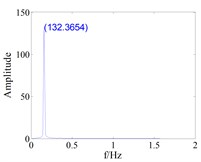b) Mistuned, ${\mathrm{k}}_{\mathrm{c}}=16$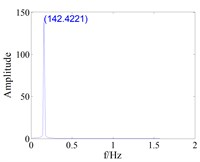c) Tuned, ${\mathrm{k}}_{\mathrm{c}}=71$d) Mistuned, ${\mathrm{k}}_{\mathrm{c}}=71$

Fig. 12The effect of tenon equivalent damping mistuning on the vibration response of blade with large gap (d0=0.8)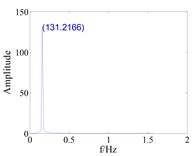a) Tuned, ${k}_{c}=\text{16}$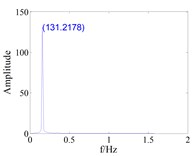b) Mistuned, ${k}_{c}=\text{16}$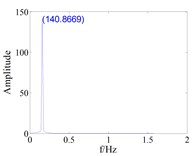c) Tuned, ${k}_{c}=\text{71}$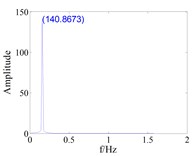d) Mistuned, ${k}_{c}=\text{71}$

### 4.3. Effect of mistuning of tenon dry friction damping force on vibration characteristics of mistuned bladed disk

In the case of different coupling strength and gap, the frequency spectrum of the coupling diagram with strong coupling stiffness ${k}_{c}=$ 71 and weak coupling stiffness ${k}_{c}=\text{16}$ are selected respectively. The gaps of 0.008 and 0.8 were selected as small and large gaps, the effect of tenon dry friction damping mistuning on the vibration response of tenon is obtained by analysis.

Fig. 13Effect of tenon’s dry friction damping force mistuning on vibration response of tenon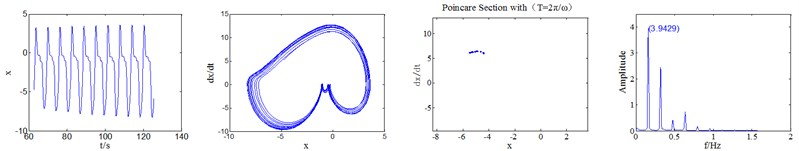a)${k}_{c}=\text{16}$, ${d}_{0}=\text{0.008}$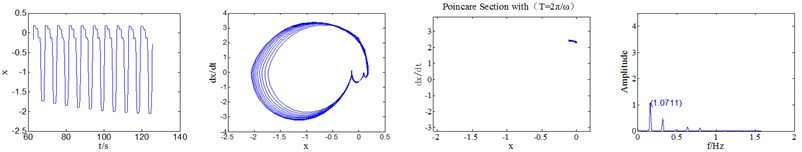b), ${d}_{0}=\text{0.008}$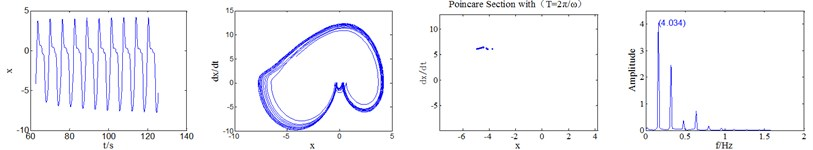c)${k}_{c}=\text{16}$, ${d}_{0}=\text{0.8}$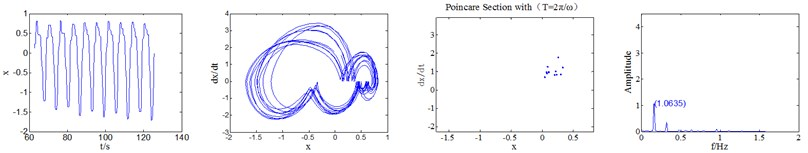d)${k}_{c}=71$, ${d}_{0}=\text{0.8}$

It can be seen from Fig. 13 that when the gap is the same, the vibration amplitude of the tenon is smaller than that of the weak coupling. When the coupling strength is strong and the gap is large, the vibration amplitude of the tenon is smaller than that the gap is small. In the case of weak coupling, when the gap is large, the vibration amplitude of the tenon is larger than that of the small gap. The gap has little effect on the vibration response of the weakly coupled bladed disk, but the gap has a great influence on the strongly coupled bladed disk, the larger the gap, the more complex the nonlinear dynamic characteristics of tenon. It can be seen from the comparison of Fig. 4 and Fig. 13 that under the same gap and coupling strength, the dry friction damping mistuning of tenon increases the vibration amplitude of tenon.

Fig. 14Response of blade vibration when tenon’s dry friction damping force mistuning under weak coupling (kc=16)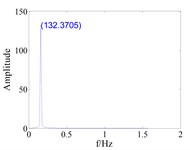a) Tuned ${\mathrm{d}}_{0}=\text{0.008}$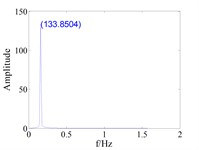b) Mistuned ${d}_{0}=0.008$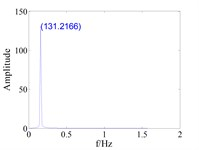c) Tuned ${\mathrm{d}}_{0}=\text{0.8}$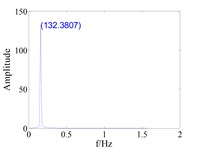d) Mistuned ${d}_{0}=0.8$

Fig. 15Response of blade vibration when tenon’s dry friction damping force mistuning under strong coupling (kc=71)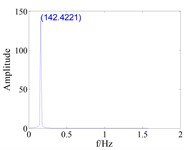a) Tuned ${d}_{0}=\text{0.008}$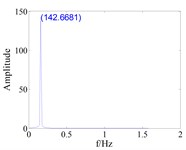b) Mistuned ${d}_{0}=\text{0.008}$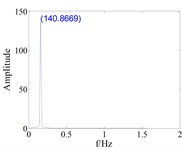c) Tuned ${d}_{0}=\text{0.8}$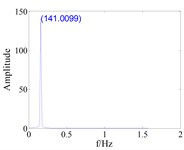d) Mistuned ${d}_{0}=\text{0.8}$

It can be seen from Fig. 14 and Fig. 15 that under the condition of the same gap, the vibration amplitude of the blade with strong coupling is larger; when the coupling stiffness and gap are the same, the tenon’s equivalent damping mistuning will increase the vibration amplitude of the blade, but the vibration amplitude of the blade will change slightly.

## 5. Conclusions

Aiming at the Tenon and mortise structure of aero-engine compressor bladed disk, a dynamic model including blade, tenon and disk was established. The nonlinear dynamic characteristics of bladed disk system with damping mistuning were studied.

1) The dynamic model and nonlinear vibration equation including blade body, tenon and disk are established. The effects of tenon gap and dry friction on the nonlinear dynamics of bladed disk are considered in the model.

2) Comparative analysis of the effects of tenon gap and dry friction on the vibration response of the mistuned bladed disk. The results show that the tenon gap and dry friction have a certain influence on the vibration response of the mistuned bladed disk, which cannot be ignored in the dynamic analysis.

3) The effects of blade equivalent damping and tenon equivalent damping mistuning on the vibration characteristics of bladed disk system are obtained: When the gap is the same, the vibration amplitude of the tenon is smaller than that of the weak coupling under the condition of strong coupling; The vibration amplitude of the tenon is smaller than that of the small gap under the condition of strong coupling; In the case of weak coupling, the vibration amplitude of tenon is larger than that of small gap when the gap is large.

4) The effects of the mistuning of the tenon’s dry friction damping force on the vibration characteristics of the mistuned bladed disk is obtained: Under the same gap, the blade vibration amplitude is larger when the coupling is strong; When the coupling stiffness and gap are the same, the equivalent damping mistuning of blade will lead to the increase of blade vibration amplitude, but the change of blade vibration amplitude is small.

#### Acknowledgements

This research is supported by project of the National Natural Science Foundation of China (No. 51805076, No. 51775093 and No. U1708255), China Postdoctoral Science Foundation (2019M651147), Doctoral Research Initiation Fund of Liaoning Provincial (2020-BS-156), Shenyang Youth Science and Technology Innovation Talent Project (RC200006) and Scientific Research Fund Project of Liaoning Provincial Department of Education (LG202008).

03 August 2020
Accepted
12 February 2021
Published
02 March 2021
SUBJECTS
Mechanical vibrations and applications
Keywords